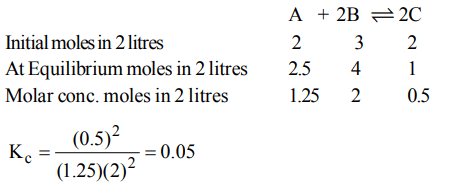## Chemical Equilibrium Questions and Answers Part-5

1. In the equilibrium reaction involving the dissociation of $CaCO_{3}$
$CaCO_{3}\left(s\right)\rightleftharpoons CaO\left(s\right)+CO_{2}\left(g\right)$
the equilibrium constant is given by
a) $\frac{p_{CaO}*p_{CO_{2}}}{p_{CaCO_{3}}}$
b) $C_{CaO}*\frac{p_{CO_{2}}}{C_{CaCO_{3}}}$
c) $\frac{p_{CaO}}{p_{CaCO_{3}}}$
d) $p_{CO_{2}}$

Explanation: Kp = $p_{CO_{2}}$ others are solids. The concentration terms for solids and liquids are taken as unity

2. Steam reacts with iron at high temperature to give hydrogen gas and $Fe_{3}O_{4}\left(s\right)$  . The correct expression for the equilibrium constant is
a) $\frac{P_{H_{2}}^2}{P_{H_{2}O}^2}$
b) $\frac{\left(P_{H_{2}}\right)^{4}}{\left(P_{H_{2}O}\right)^{4}}$
c) $\frac{\left(P_{H_{2}}\right)^{4}\left[Fe_{3}O_{4}\right]}{\left(P_{H_{2}O}\right)^{4}\left[Fe\right]}$
d) $\frac{\left[Fe_{3}O_{4}\right]}{\left[Fe\right]}$

Explanation:3. In lime kiln, the reversible reaction
$CaCO_{3}\left(s\right)\rightleftharpoons CaO\left(s\right)+CO_{2}\left(g\right)$
proceeds to completion because
a) of high temperature
b) $CO_{2}$ escaped out
c) CaO is removed
d) of low temperature

Explanation: Forward reaction is favoured by removal of products

4. If 1.0 mole of $I_{2}$ is introduced into 1.0 litre flask at 1000 K, at quilibrium $\left(K_{C}=10^{-6}\right)$  , which one is correct
a) $\left[I_{2}\left(g\right)\right]>\left[I^{-}\left(g\right)\right]$
b) $\left[I_{2}\left(g\right)\right]<\left[I^{-}\left(g\right)\right]$
c) $\left[I_{2}\left(g\right)\right]=\left[I^{-}\left(g\right)\right]$
d) $\left[I_{2}\left(g\right)\right]=\frac{1}{2}\left[I^{-}\left(g\right)\right]$

Explanation:5. At equilibrium, if$K_{P}=1$  , then
a) $\triangle G^{\circ}=0$
b) $\triangle G^{\circ}>1$
c) $\triangle G^{\circ}<1$
d) None of these

Explanation:6. Van't Hoff's equation giving the effect of temperature on chemical equilibrium is represented as
a) $\frac{d In F}{dT}=\frac{\triangle H}{RT^{2}}$
b) $\frac{d ln K_{P}}{dT}=\frac{\triangle HT^{2}}{R}$
c) $\frac{d ln K_{P}}{dT}=\frac{\triangle H}{RT^{2}}$
d) $\frac{d ln K_{P}}{dT}=\frac{RT^{2}}{\triangle H}$

Explanation:7. Solubility of a substance which dissolves with a decrease in volume and absorption of heat will be favoured by
a) high P and high T
b) low P and low T
c) high P and low T
d) low P and high T

Explanation: high P and high T

8. In what manner will increase of pressure affect the following equation? $C\left(s\right)+H_{2}O\left(g\right)\rightleftharpoons CO\left(g\right)+H_{2}\left(g\right)$
a) Shift in the forward direction
b) Shift in the reverse direction
c) Increase in the yield of hydrogen
d) No effect

Explanation: Reverse reaction, Le Chatelier's principle $\triangle$n=2 – 1 = 1.

9. In a reaction, $A + 2B\rightleftharpoons 2C$    , 2.0 mole of 'A', 3.0 mole of 'B' and 2.0 mole of 'C' are placed in a 2.0 L flask and the equilibrium concentration of 'C' is 0.5 mole/L. The equilibrium constant (K) for the reaction is
a) 0.073
b) 0.147
c) 0.05
d) 0.026$H_{2}\left(g\right)+I_{2}\left(g\right)\rightleftharpoons 2HI\left(g\right)$